# transformer-XL与XLNet笔记

2019/11/11

## Transformer-XL

### 概览

Transformer编码固定长度的上下文。具体地，将一个长文本序列截断为几个固定长度的片段(segment)，然后分别编码每个片段，片段间没有任何的信息交互(如BERT的预训练模型中序列长度的极限为512)。如下图所示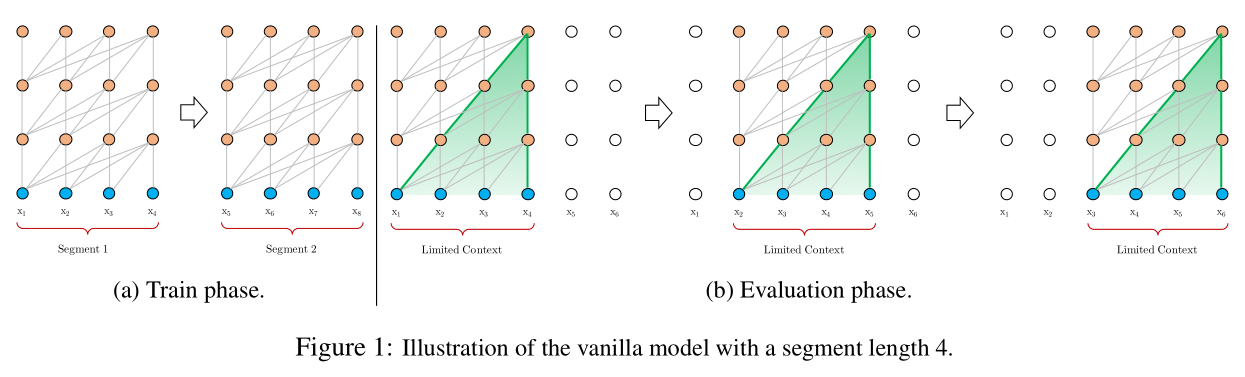• 对超过固定长度的依赖关系无法建模编码
• 对长文本的分割破坏了语义边界，导致上下文碎片化(context fragmentation)

• 片段级的递归机制(Segment-Level Recurrence with State Reuse)，引入memory模块(cache之前一个或多个segment的隐状态信息)，循环建模片段间的联系
• 使超长距离依赖关系的编码成为可能
• 使得片段之间产生交互，解决了上下文碎片化问题
• 相对位置编码(Relative Positional Encodings)，代替绝对位置编码
• 避免了memory中缓存的片段的位置信息与当前片段中的位置信息相互混淆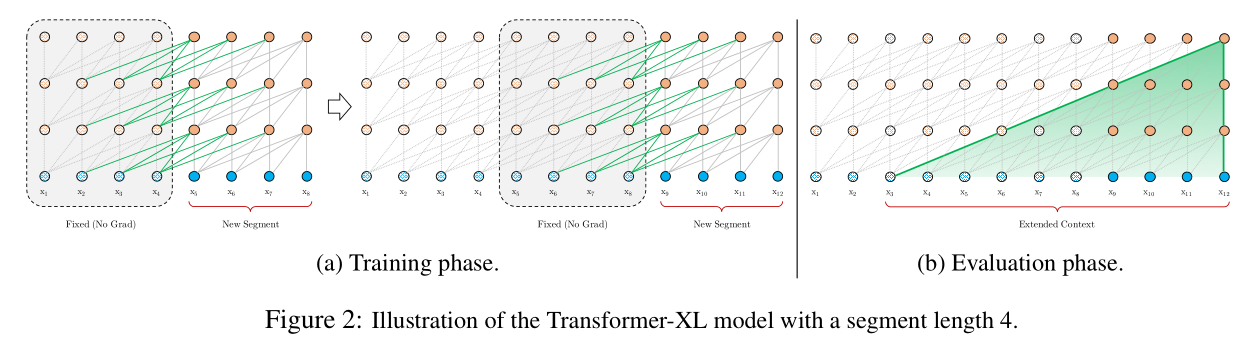### Relative Positional Encodings

recurrent机制使得先前的绝对位置编码方案不再适用，因为在多个segment中会出现多个同样的位置信息。 为此，作者们提出一种新的相对位置编码形式。其不仅与绝对位置一一对应，而且具有更好的泛化性。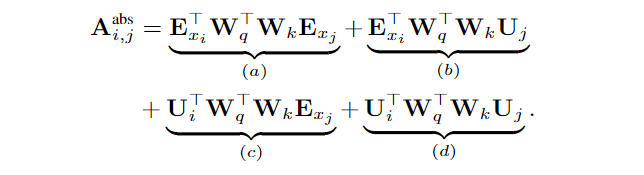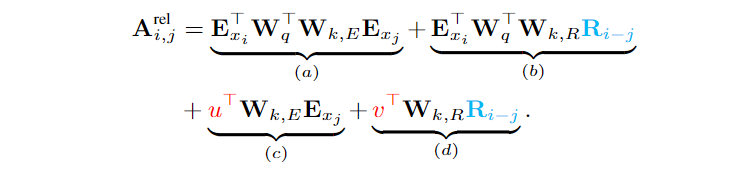• $\left( b \right)$$\left( d \right)$项的绝对位置信息$U_j$替换成相对位置信息$R_{i-j}$。这在本质上反映了一个先验：只有相对距离才是主要的关注点。其中$R$是像transformer中那种不可学习的正弦编码矩阵。该种编码使得在一定长度的memory上训练的模型可以自动推广到评估期间要长好几倍的memory上

• $\left( c \right)$$\left( d \right)$项的$U_{i}^{T}W_{q}^{T}$分别替换成可学习的向量$u\in\mathbb{R}^d$$v\in \mathbb{R}^d$。将$U_{i}^{T}W_{q}^{T}$替换成可学习的向量，表明对于所有的query位置对应的query(位置)向量是相同的。 即无论query位置如何，对不同词的注意偏差都保持一致。

• 将转换key的权重矩阵$W_k$分成基于内容的key的权重矩阵$W_{k,E}$和基于位置的key的权重矩阵$W_{k,R}$

• $\left( a \right)$表示基于内容的寻址，即没有考虑位置编码的原始分数
• $\left( b \right)$表示内容相关的位置偏差，即相对于当前内容的位置偏差
• $\left( c \right)$表示全局的内容偏置，从内容层面衡量键的重要性
• $\left( d \right)$表示全局的位置偏置，从相对位置层面衡量键的重要性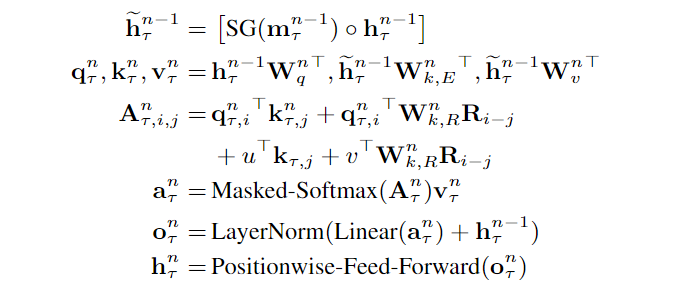### 结论

transformer-XL是一种强大的语言模型。其有较低的困惑度，与RNN和Transformer相比，可建模更长的依赖关系，在评估过程中实现了实质性的加速，并能够生成连贯的文章。

## XLNet

### AR与AE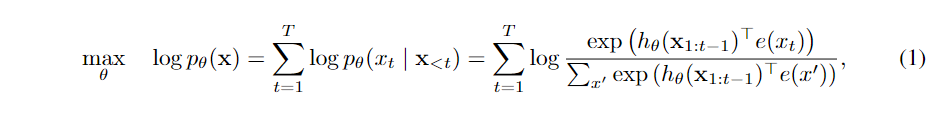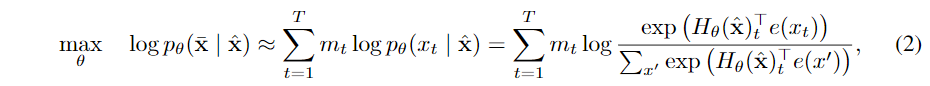• 独立假设。BERT基于所有被mask的token间是相互独立的假设对联合条件概率$p\left( \bar{\text{x}}\left\| \hat{\text{x}} \right. \right)$进行因式分解的。式$\left( 2 \right)$中的$\approx$ 强调了这里有独立假设导致等号不成立。而式$\left( 1 \right)$中则没有这种假设。

• 输入噪声。式$\left( 2 \right)$引入了mask，使得预训练与下游任务不一致。而式$\left( 1 \right)$中则没有这种输入噪声。(此处虽然在原始输入中没有直接引入噪声，但是在内部处理的时候会用到掩码矩阵进行token的预测)

• 上下文的依赖。式$\left( 1 \right)$只能依赖当前位置左边的token，而$\left( 2 \right)$可同时依赖左右两边的token。 这使得bert在NLU方面的性能要好于GPT

### 排列语言模型(Permutation Language Model)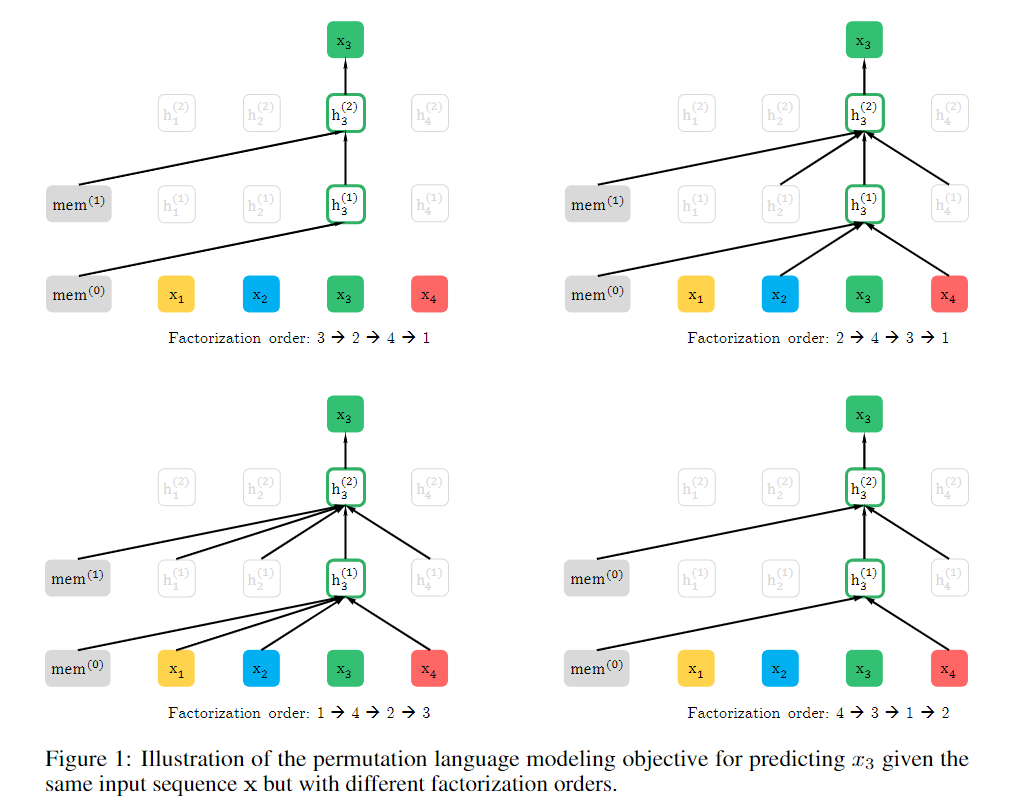### Two-Stream Self-Attention for Target-Aware Representations#### Two-Stream Self-Attention

• content表示$h_{\theta}\left( \text{x}_{\text{z}\le t} \right)$，简写为$h_{z_t}$。与传统transformer中的隐状态相似，其可编码$x_{z_t}$的上(下)文$% $$x_{z_t}$自身。图示说明如下：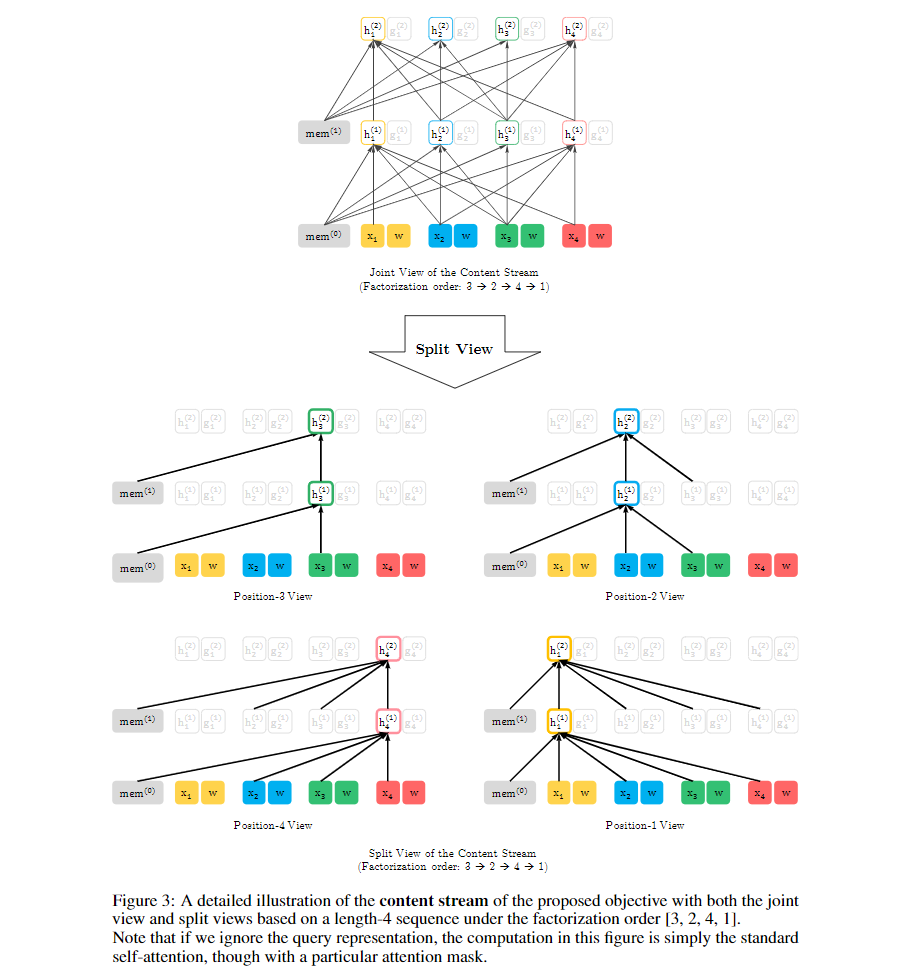• query表示$% $，简写为$g_{z_t}$。其可编码$x_{z_t}$的上(下)文$% $$x_{z_t}$的位置信息，不能编码$x_{z_t}$的内容(content)。图示说明如下：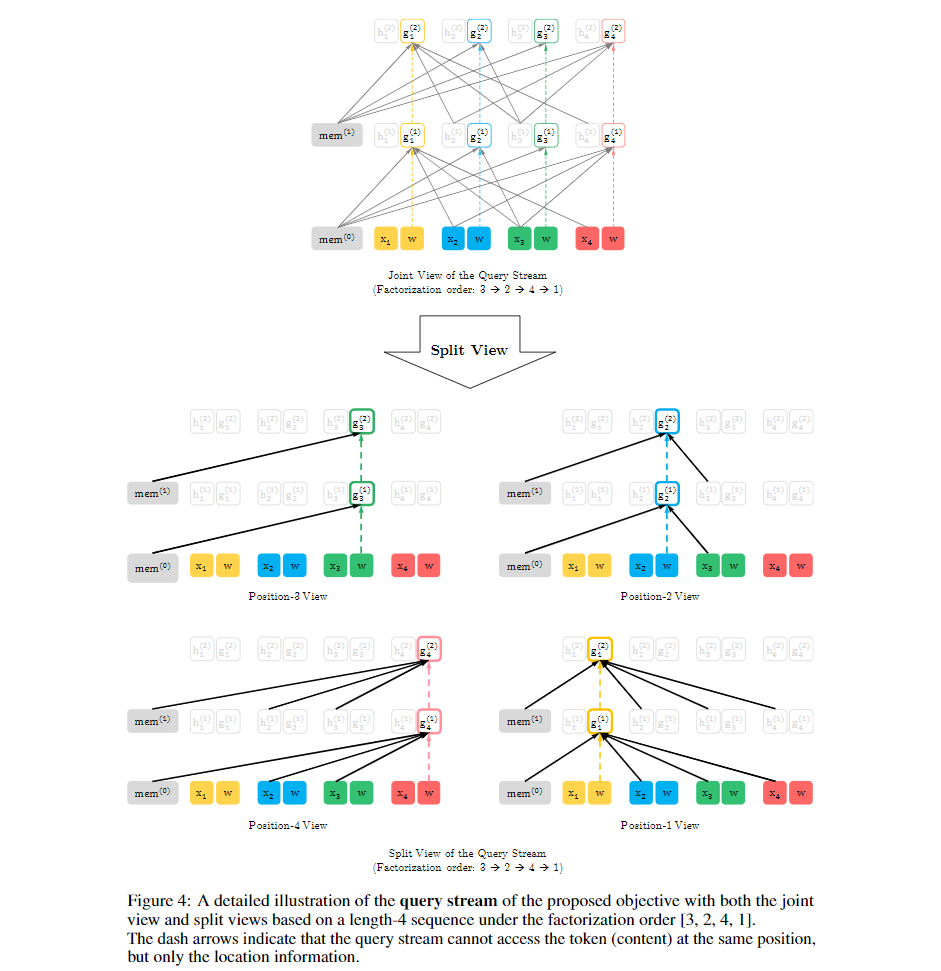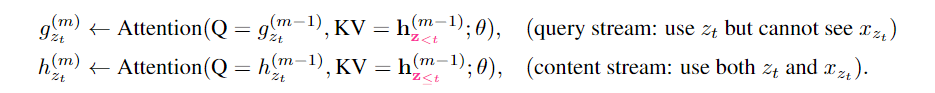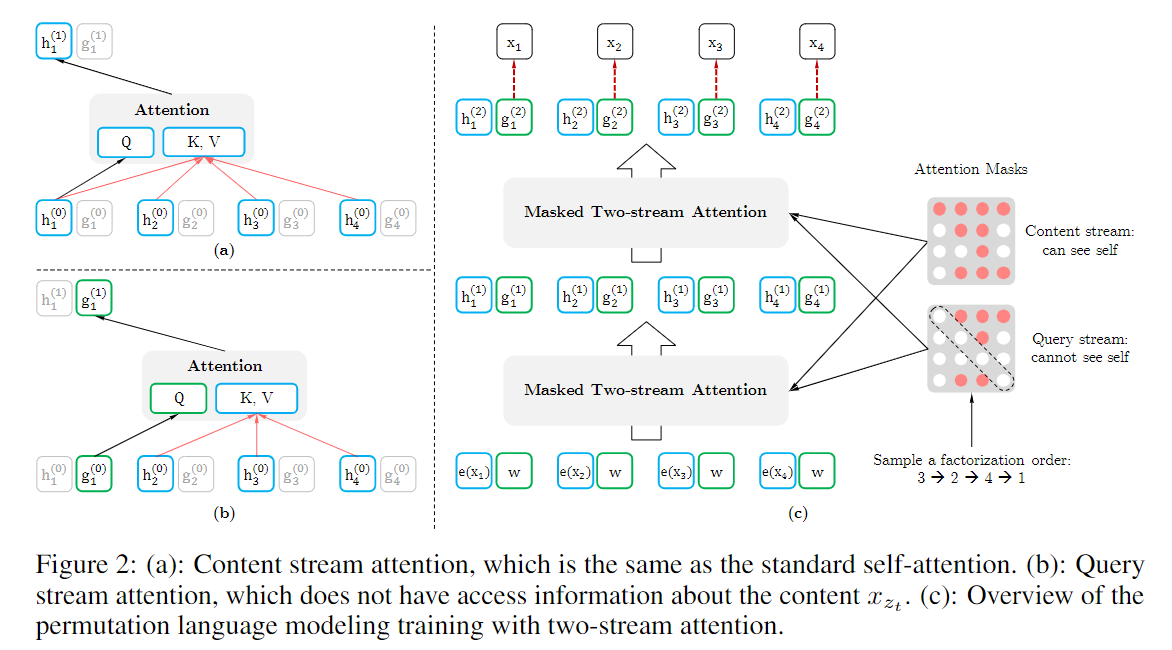#### Partial Prediction### Incorporating Ideas from Transformer-XLquery stream的表示与上述类似。图2$\left( c \right)$及图3、图4展示了基于双流自注意机制的PLM的概述。